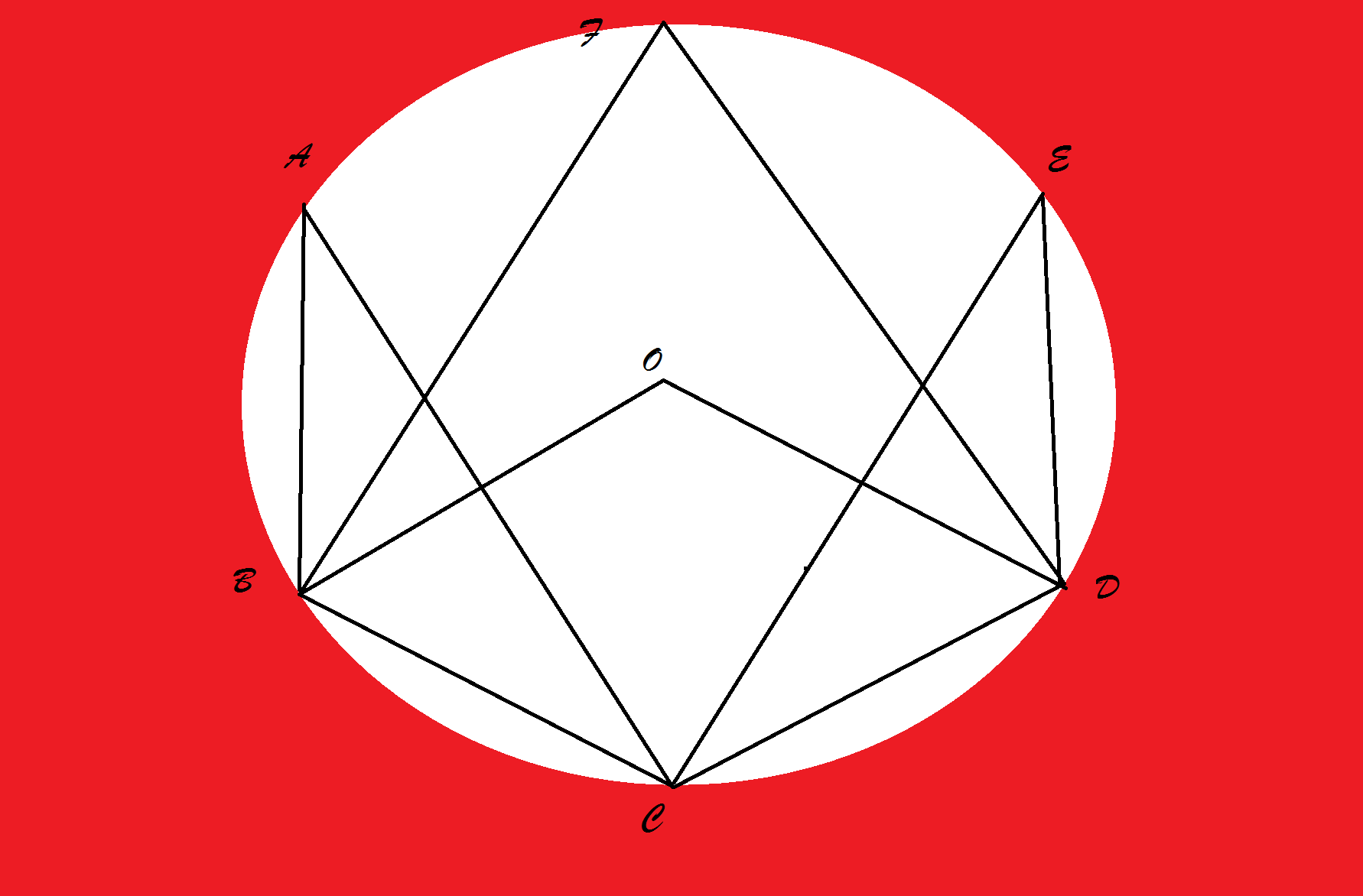# Properties of circle................

Geometry Level 3In the given figure above O is the centre of the circle, BC and CD are two equal chords and $\angle OBC = 70 ^ \circ$. You have to find sum of $\angle BAC+\angle BFD+\angle CED$(in degrees).

×